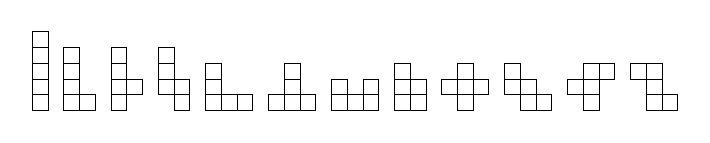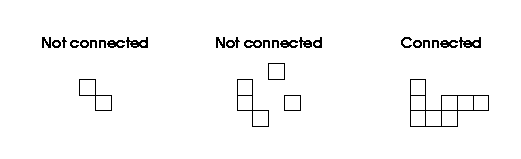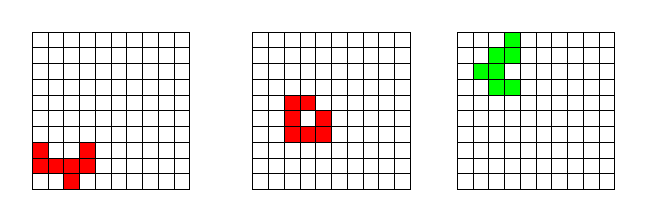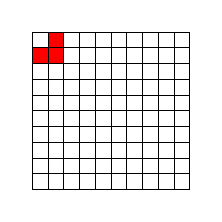### Problem Statement

You are given a rectangular board consisting of width times height small squares. Return the number of different ways there are to cut a k-polyomino out of this board, so that the remaining part remains connected.

A k-polyomino is a connected set of k squares. The figure below shows as an example all possible pentominoes (5-polyominoes) ignoring any translations, rotations or reflections.Connected means side-connected here (both in the polyomino definition and in the requirement for the remaining part of the board) - i.e., you must be able to go from any square to any other by only going through squares and square-sides but not square-corners.For two ways of cutting a polyomino out of the grid to be different and be counted separately it suffices if at least one grid square is included in the first polyomino but not in the other.

The example below shows some polyomino cuts for k=7. The two on the left (red) are not counted, since they leave the remaining grid disconnected, while the one on the right (green) is counted.### Definition

 Class: PolyominoCut Method: count Parameters: int, int, int Returns: int Method signature: int count(int k, int width, int height) (be sure your method is public)

### Constraints

-k will be between 1 and 8, inclusive.
-width and height will be between k+1 and 800, inclusive.

### Examples

0)

 `1` `10` `20`
`Returns: 200`
 There is only one 1-polyomino (called monomino), and this can be cut out anywhere on the grid.
1)

 `3` `10` `10`
`Returns: 480`
 There are in total 484 ways to cut a triomino (3-polyomino) out of a 10x10 square, but 4 of them leave a square in the corner not connected (see figure below), so only 480 satisfy the problem requirements.2)

 `8` `800` `800`
`Returns: 1704053350`
 The worst case fits in an int.

#### Problem url:

http://www.topcoder.com/stat?c=problem_statement&pm=4489

#### Problem stats url:

http://www.topcoder.com/tc?module=ProblemDetail&rd=7217&pm=4489

gepa

#### Testers:

PabloGilberto , lbackstrom , brett1479

#### Problem categories:

Graph Theory, Recursion, Simple Math